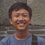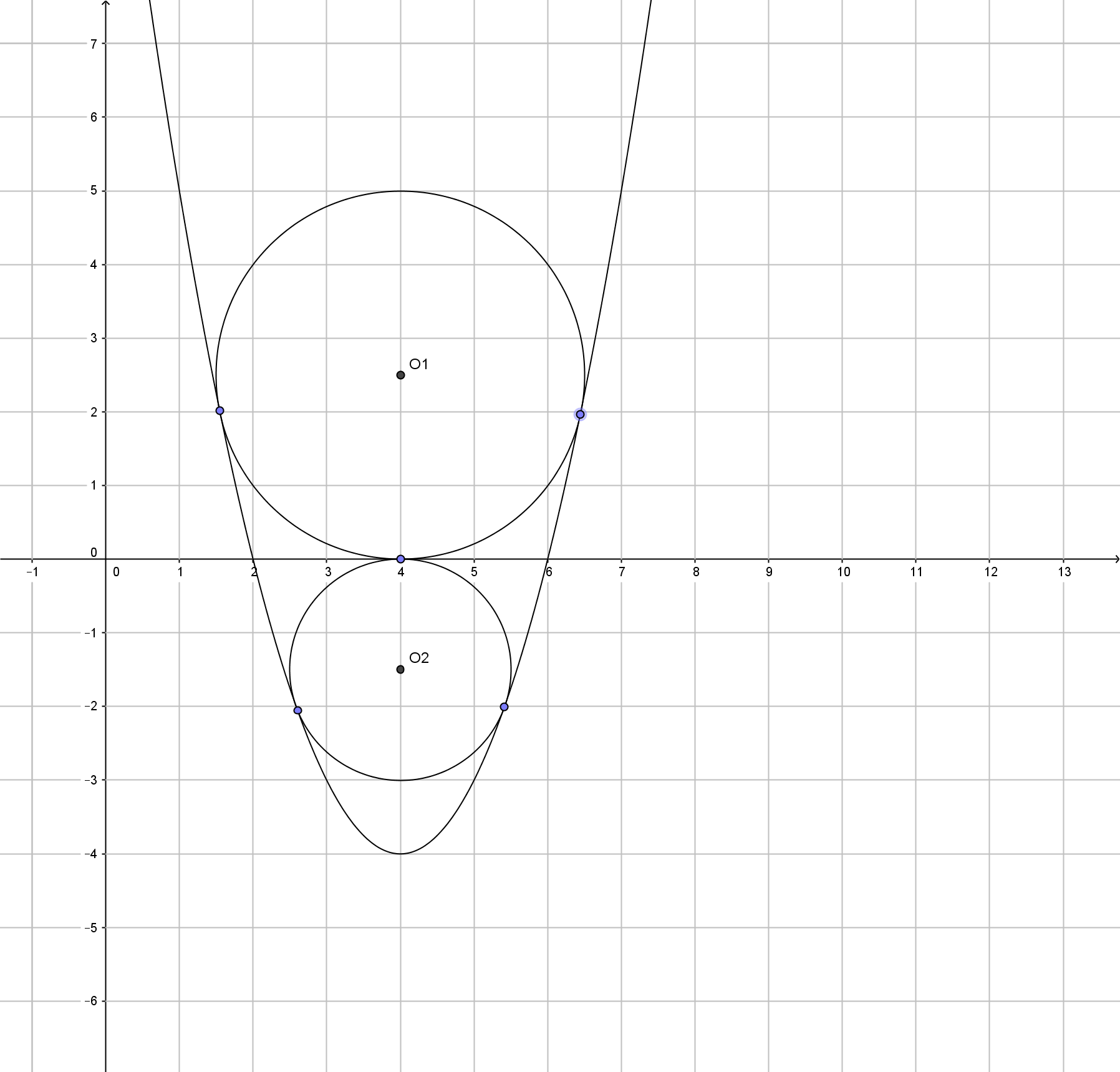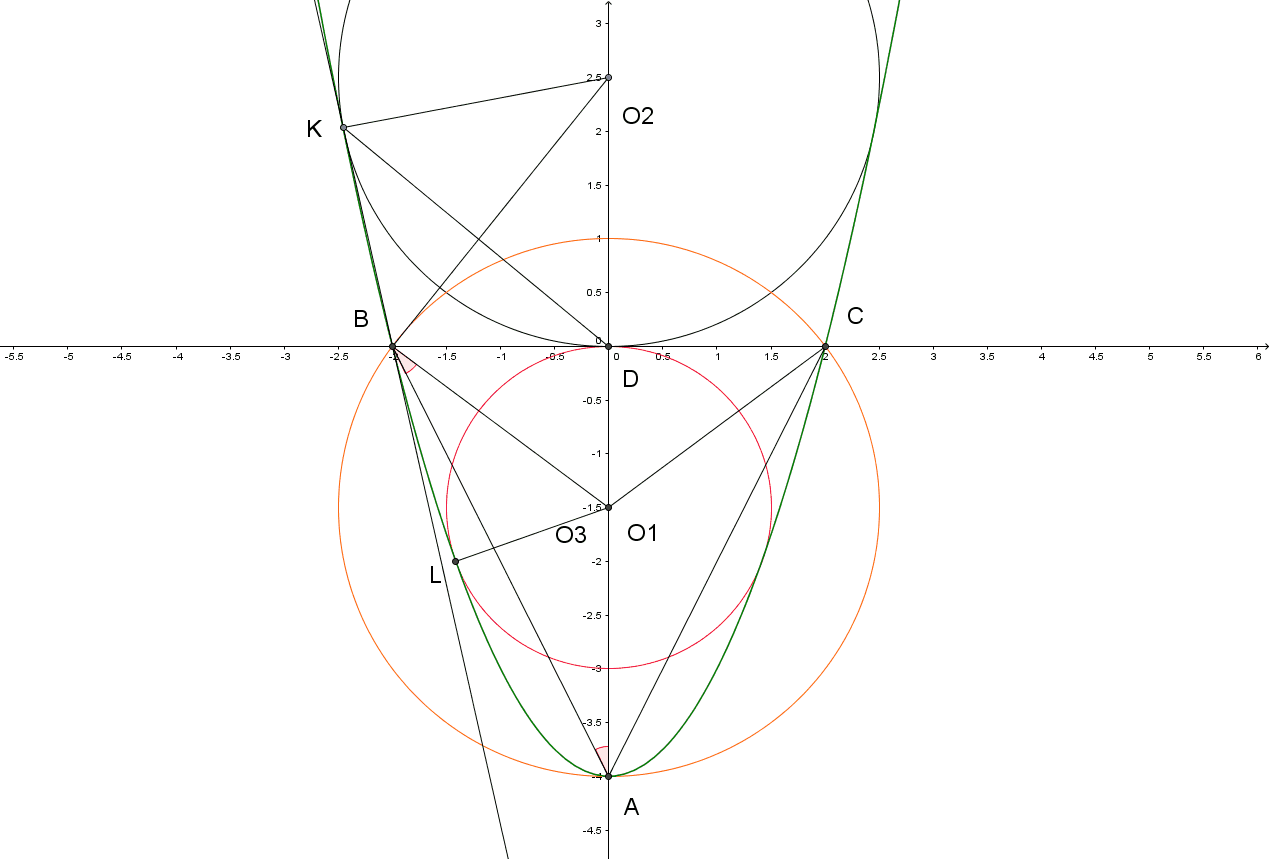# Anyone help !!!

I found this problem was very difficult :(. Can anyone help me solve this? Here is the problem:

Given a parabola $y = x^2 - 8x + 12$. Find the ratio of two inscribed circle on the parabola, both bordered by the parabola and the $x$ axis. But one circle is over $x$ axis and the other one is under $x$ axis.Note by Muh Amin
6 years ago

This discussion board is a place to discuss our Daily Challenges and the math and science related to those challenges. Explanations are more than just a solution — they should explain the steps and thinking strategies that you used to obtain the solution. Comments should further the discussion of math and science.

When posting on Brilliant:

• Use the emojis to react to an explanation, whether you're congratulating a job well done , or just really confused .
• Ask specific questions about the challenge or the steps in somebody's explanation. Well-posed questions can add a lot to the discussion, but posting "I don't understand!" doesn't help anyone.
• Try to contribute something new to the discussion, whether it is an extension, generalization or other idea related to the challenge.

MarkdownAppears as
*italics* or _italics_ italics
**bold** or __bold__ bold
- bulleted- list
• bulleted
• list
1. numbered2. list
1. numbered
2. list
Note: you must add a full line of space before and after lists for them to show up correctly
paragraph 1paragraph 2

paragraph 1

paragraph 2

[example link](https://brilliant.org)example link
> This is a quote
This is a quote
    # I indented these lines
# 4 spaces, and now they show
# up as a code block.

print "hello world"
# I indented these lines
# 4 spaces, and now they show
# up as a code block.

print "hello world"
MathAppears as
Remember to wrap math in $$ ... $$ or $ ... $ to ensure proper formatting.
2 \times 3 $2 \times 3$
2^{34} $2^{34}$
a_{i-1} $a_{i-1}$
\frac{2}{3} $\frac{2}{3}$
\sqrt{2} $\sqrt{2}$
\sum_{i=1}^3 $\sum_{i=1}^3$
\sin \theta $\sin \theta$
\boxed{123} $\boxed{123}$

Sort by:

the length of the circumference O1 = 15.71(approximately)

the length of the circumference O2 = 9.43(approximately)

Co1/Co2 = 1.7

Calculations made with Geogebra- 6 years ago

Is there no other 'common' way to solve this problem? I mean without using software? I just want to know how to solve this problem. But firstly, thank you so much for your help.

- 5 years, 12 months agoI don't know English very well, but I'll try to help you :

First of all let's rewrite our function in this form (it will be the same result at the end since I am using Translation (geometry)):

$f(x)=x^{2}-4$

Then we must find the coordinates of the vertex of our new parabola and roots of $x^{2}-4=0$

Here it is :

1) coordinates of the vertex : $x=0$ and $y=-4$ ,so : $(0;-4)$

2) roots : $x = \pm2$ , also we have two points : $(-2;0)$ and $(2;0)$

So, now we should define some points (take a look at my picture) : $A (0;-4)$ , $B (-2;0)$ , $C (2;0)$ also let's suppose that $O1$ and $O2$ are the centres of our circles, where $O1(red) (0;y)$ and $O2 (0;y1)$ .

Let's take a look at triangle $ABC$ :

$AB=AC = \sqrt { 20 }$(by Pythagorean theorem) and ${ S }_{ \Delta ABC } = 8$

Let's draw circumcircle $O3$(orange) of the triangle $ABC$ and find it's radius : $R=\frac { AB*BC*CA }{ 4{ S }_{ ABC } }$, where $R= 2.5$ .

So, the centre of $O3$ is obviously point $(0;-1.5)$

/**********************************************************

Let's suppose that point $(0;-1.5)$ is also the centre of circle $O1$. It means, that $R(O1)=1.5$.

To prove that point $(0;-1.5)$ is the centre of $O1$ we must prove that line segment $LO1=1.5$.

Straight line $LO1$ is a $normal$- line that is perpendicular to our parabola in point $L$.

So, here it's function : $f(x)=-\frac { 1 }{ 2{ x }_{ 0 } } (x-{ x }_{ 0 })+({ { x }_{ 0 } }^{ 2 }-4)$ (${ x }_{ 0 }$ is the touch abscissa(point $L$) of the parabola and circle $O1$)

Let's find ${ x }_{ 0 }$. Under our assumption point $O1$ has the following coordinates $(0;-1.5)$. Now we must put them in our $normal$ function.

$-\frac { 1 }{ 2{ x }_{ 0 } } (0-{ x }_{ 0 })+({ { x }_{ 0 } }^{ 2 }-4)=-1.5$

Where ${ x }_{ 0 }=-1.414213562$. Now we must put ${ x }_{ 0 }$ in the parabola function.

$y={(-1.414213562) }^{ 2 }-4$ , $y=-2$. Ordinate of point $L=-2$. So, $L=(-1.414213562;-2)$

Now, we should check if $|LO1|=|O1D|=1.5$

$|LO1|=\sqrt { { (0-(-1.414213562)) }^{ 2 }+{ (-1.5-(-2)) }^{ 2 } } = 1.5$.

Our assumptions were confirmed =) . So point $(0;-1.5)$ is the centre of circle $O1$. And of course $|O1D|={ R }_{ O1 }=1.5$

/**********************************************************

Now, the next part of my horrible solution.

It's much easier to find the centre of $O2$.

Let's assume that the straight line $KB$ coincides with our parabola ($y\in (0;6)$) and tangent line to the parabola at point $B(-2;0)$

So, we can find $\tan { (\angle KBD) }$

Firstly, let's find the tangent line function : it's $f(x)'=({ x }^{ 2 }-4)'=2x$ then put abscissa of point $B$ in this function.

So, $f'(-2)=-4=\tan { \angle } KBD \Rightarrow { \angle } KBD\approx { 104 }^{ \circ }$

Since $KB$ and $BD$ are tangents to the circle $O2$ $\Rightarrow$ $KB=BD$. Also $KO2=O2D$ $\Rightarrow$ $O2B$-bisector and median $\Rightarrow$ $O2BD\approx { 52 }^{ \circ }$

$\tan { \angle } O2BD\approx 1.28$ $\Rightarrow$ $1.28=\frac { O2D }{ DB } =\frac { O2D }{ 2 }$ $\Rightarrow$ $O2D=2.5$

$O2=(0;2.5)$ $\Rightarrow$ ${ R }_{ O2 }=2.5$

/**********************************************************

And of course the last step :

$\frac { { R }_{ O1 } }{ { R }_{ O2 } } =0.6$

That's it $=)$

- 5 years, 12 months ago

Thank you very much Fatum. My english is not very good also :)

- 5 years, 12 months ago

But why exactly this problem, why did you want to solve it?

- 5 years, 12 months ago

I just randomly solve problem from my book. And from those problems, I just can't solve this one

- 5 years, 11 months ago

What book?

- 5 years, 11 months ago

Text book. I'm a student of high school

- 5 years, 11 months ago

Both the circles touch X axis at (4,0) by symmetry.We can interpret this as follows -these two circles are the members of family of circles that pass through through the intersection of line y=0 and the circle with centre (4,0) and radius 0.We know that S+ku=0 represents family of circles passing through intersection of circle S=0 and line u=0 where k is arbitrary parameter.Now solve the equation of circle and parabola to obtain a quadratic equation in y.Since the parabola and circle touch each other set the discriminant of the quadratic as 0.This will give two values of k namely 3 and -5.The two values of k give us the two equations of the circles.

- 5 years, 10 months ago